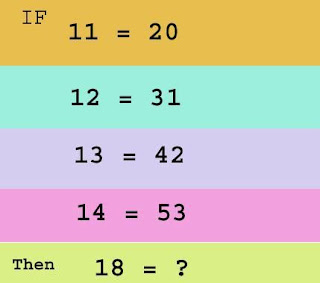Gudang Info yang berisi Berita, Cerita, Hiburan, dan Ilmu Pengetahuan

Logic and Proofs Discrete Mathematics-Resume

PROPOSITIONAL LOGIC
A proposition is a declarative sentence (that is, a sentence that declares a fact) that is either true or false, but not both.
a.      The negation of p, denoted by ¬p (also denoted by p), is the statement “It is not the case that p.” The proposition ¬p is read “not p.” The truth value of the negation of p, ¬p, is the opposite of the truth value of p.
b.      The conjunction of p and q, denoted by p q, is the proposition “p and q.” The conjunction p q is true when both p and q are true and is false otherwise.
c.       The disjunction of p and q, denoted by p q, is the proposition “p or q.” The disjunction p q is false when both p and q are false and is true otherwise.
d.      The XOR (exclusive or) of p and q, denoted by p q, is the proposition that is true when exactly one of p and q is true and is false otherwise.
e.       The implication of p → q is the proposition “if p, then q.” The conditional statement p → q is false when p is true and q is false, and true otherwise.
f.       The bi-implications statement p ↔ q is the proposition “p if and only if q.” The statement p ↔ q is true when p and q have the same truth values, and is false otherwise.

Logic and Proofs
Discrete Mathematics
Resume”fropky.com

MATHEMATICAL PROOFS
A proof is a valid argument that establishes the truth of a mathematical statement. A proof can use the hypotheses of the theorem, if any, axioms assumed to be true, and previously proven theorems.
a.      Direct Proofs. A direct proof of a conditional statement p → q is constructed when the first step is the assumption that p is true; subsequent steps are constructed using rules of inference, with the final step showing that q must also be true. A direct proof shows that a conditional statement p → q is true by showing that if p is true, then q must also be true, so that the combination p true and q false never occurs.

b.      Proofs by Contradiction. Another type of indirect proof. Suppose we want to prove that a statement p is true. The contradiction q such that ¬p → q is true. Because q is false, but ¬p → q is true, we can conclude that ¬p is false, which means that p is true. Because the statement r ¬r is a contradiction whenever r is a proposition, we can prove that p is true if we can show that ¬p → (r ¬r) is true for some proposition r.

c.       Proof by Contraposition. Another type of indirect proof. Use of the fact that the conditional statement p → q is equivalent to its contrapositive, ¬q →¬p. This means that the conditional statement p → q can be proved by showing that its contrapositive, ¬q →¬p, is true. In a proof by contraposition of p → q, we take ¬q as a premise, and using axioms, definitions, and previously proven theorems, together with rules of inference, we show that ¬p must follow.
Reference: Discrete_Mathematics_and_Its_Applications_7th_Edition_Rosen
For full document